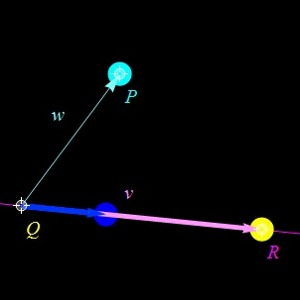## What are scalar and vector projections?

Here are the definition. In order to understand something one always has to know the definitions first:

 Pw(v) = (v.w) w/|w|2 vector projection Cw(v) = (v.w)/|w| scalar projectionThe vector projection is a vector parallel to w. The scalar projection is a scalar. If the angle between v and w is smaller than 90 degrees, then the scalar projection is the length of the vector projection. If the angle is larger than 90 degrees then the scalar projection is minus the length of the vector projection.

## What is the significance of the scalar and vector projections?

The vector projection is useful in physics, especially with forces. If an object moves with velocity v and F is a force, then the projection of F onto v gives the accelation in the direction v. onto w is the force which is relevant.
The scalar projection is a scalar. It is positive if the angle between the vectors is acute, it is negative if the angle is negative. It is zero if the angle is a right angle.
The scalar projection allows to visualize the dot product. If w has length 1, then the scalar projection of v onto w is the dot product. The scalar projection is also useful for distances. The distance between a point P and a plane through a point Q with normal vector n is the scalar projection of PQ onto n.

## How can one visualize the projection?

A Mathematica demonstration

## Can it be generalized ?

Yes, one can define projection onto any linear space. This is done in linear algebra. For those of you who have already seen linear algebra: if A is the matrix which contains vectors spanning a space V as column vectors, then the projection onto this space V is
``` P(v) =  A (AT A)-1 AT  v
```
This is extremely useful, for example for data fitting in statistics. If A=[w] is a column vector, then (AT A) = |w|^2 and AT v = (w.v) and A = w so that we recover the above formula as a special case.